+

Author: Sophia Tutorial
##### Description:

(more)

Sophia’s self-paced online courses are a great way to save time and money as you earn credits eligible for transfer to many different colleges and universities.*

No credit card required

37 Sophia partners guarantee credit transfer.

299 Institutions have accepted or given pre-approval for credit transfer.

* The American Council on Education's College Credit Recommendation Service (ACE Credit®) has evaluated and recommended college credit for 32 of Sophia’s online courses. Many different colleges and universities consider ACE CREDIT recommendations in determining the applicability to their course and degree programs.

Tutorial
what's covered

Not all numbers have a nice even square root. For example, if we found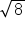on our calculator, the answer would be 2.828427124746190097603377448419... and even this number is a rounded approximation of the square root. Decimal approximations are good estimates, but you may need the exact value. To do this, we will simplify radical expressions using the product property of square roots:

formula
Product Property of Square Roots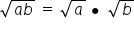We can use the product rule to simplify an expression such as √180 by splitting it into two roots, √36 . √5, and simplifying the first root, 6√5. The trick in this process is being able to translate a problem like √180 into √36 • √5. There are several ways this can be done.

hint
The most common and, with a bit of practice, the fastest method, is to find perfect squares that divide evenly into the radicand, or number under the radical. This is shown in the next example.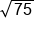75 is divisible by 25, a perfect square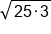Split into factors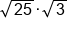Product rule, take the square root of 25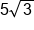Our Solution

If there is a coefficient in front of the radical to begin with, the problem merely becomes a big multiplication problem.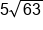63 is divisible by 9, a perfect square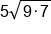Split into factors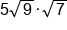Product rule, take the square root of 9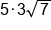Multiply coefficients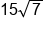Our Solution

As we simplify radicals using this method it is important to be sure our final answer can be simplified no more.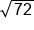72 is divisible by 9, a perfect square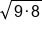Split into factors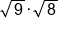Product rule, take the square root of 9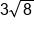But 8 is also divisible by a perfect square, 4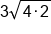Split into factors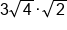Product rule, take the square root of 4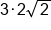Multiply

The previous example could have been done in fewer steps if we had noticed that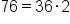, but often the time it takes to discover the larger perfect square is more than it would take to simplify in several steps.

We can simplify higher roots in much the same way we simplified square roots, using the product property of radicals.

formula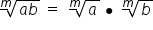Often we are not as familiar with higher powers as we are with squares. It is important to remember what index we are working with as we try and work our way to the solution.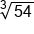We are working with a cubed root, want third powers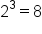Test 2,, 54 is not divisible by 8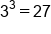Test 3,, 54 is divisible by 27!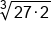Write as factors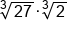Product rule, take cubed root of 27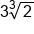Our Solution

Just as with square roots, if we have a coefficient, we multiply the new coefficients together.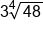We are working with a fourth root, want fourth powers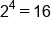Test 2,, 48 is divisible by 16!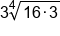Write as factors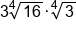Product rule, take fourth root of 16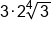Multiply coefficients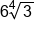Our Solution

summary
We can use the product or quotient properties to combine or break down radicands through multiplication or division and this will help us simplify radical expressions. When we're combining two radicals into one using the product or quotient property, the index of the radicals must be the same.

Source: Adapted from "Beginning and Intermediate Algebra" by Tyler Wallace, an open source textbook available at: http://wallace.ccfaculty.org/book/book.html

Formulas to Know